# How to use the DAYS function in Excel

In simple words, DAYS function returns the number of days between 2 dates in excel

DAYS function gets a number which is count of days between two given dates.
Syntax:

=DAYS (end_date, start_date)

Start_date : from the date
End_date : till the date

Let’s understand this function using it in an example.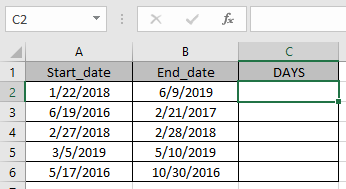Use the formula.

B2 : end_date
A2 : start_date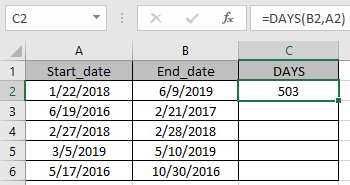You can see the formula returns a number, the total days elapsed between the two dates.

Now copy the formula to other cells using shortcut key Ctrl + D.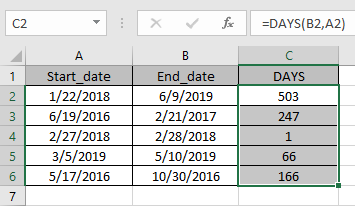Use the above function formulas to get the days between dates in Excel.

Alternate method

Excel stores dates as numbers, a simple mathematical formulation explained. So just need to subtract the two dates it will get the days between the two dates.
<div="bbbb">=later_date - earlier date

Or

=IF(date1>date2, date1 - date2, date2 - date1)

Use the below formula, if you don’t know which date to put first.
Let’s understand this function using it an example.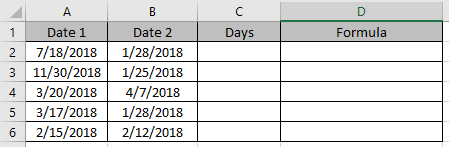Use the formula in C2 and D2 cells.

=A2-B2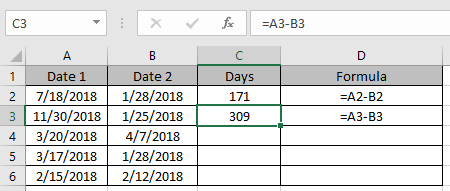This formula gets you negative results if the date1 < date2.
If you don’t want to care which is smaller, just use the below formula in remaining cells

=IF(A4>B4, A4-B4, B4-A4)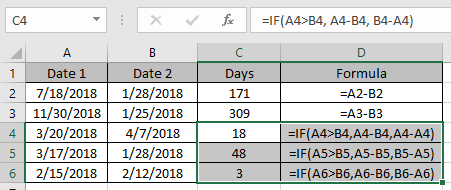Use the above function formulas to get the days between dates in Excel

Hope you understood how to get the days between dates using DAYS function in Excel. Explore more articles on Excel Date & TIME functions here. Please feel free to state your query or feedback for the above article.

Top Articles:

Popular Articles:

50 Excel Shortcuts to Increase Your Productivity

How to use the VLOOKUP Function in Excel

How to use the COUNTIF in Excel 2016

How to use the SUMIF Function in Excel

## Users are saying about us...

1.How can i re-name rows (1,2,3,..) and columns (a,b,c,..) to a custom setting

2."hi
dear sir,
i just want to know that is it any formula which can we delete duplicate record in selected range of cell's and restrict a cell does not take duplicate records.
Thanks"

Terms and Conditions of use

The applications/code on this site are distributed as is and without warranties or liability. In no event shall the owner of the copyrights, or the authors of the applications/code be liable for any loss of profit, any problems or any damage resulting from the use or evaluation of the applications/code.